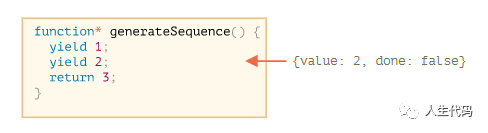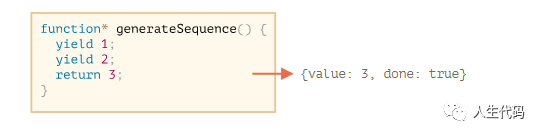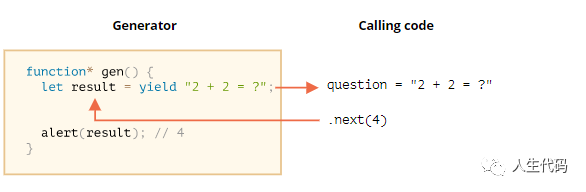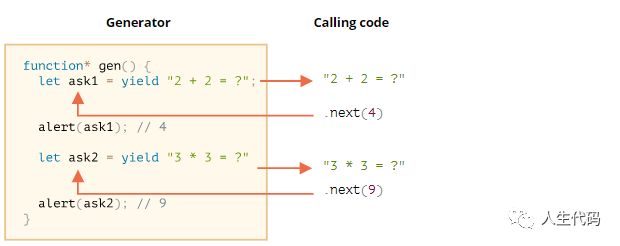03/01 19:46

# 生成器函数

function* generateSequence() {  yield 1;  yield 2;  return 3;}

function* generateSequence() {  yield 1;  yield 2;  return 3;}// "generator function" creates "generator object"let generator = generateSequence();alert(generator); // [object Generator]next()的结果总是一个具有两个属性的对象:

• value:生成的值。
• done:如果函数代码已经完成，则为 true，否则为 false

function* generateSequence() {  yield 1;  yield 2;  return 3;}let generator = generateSequence();let one = generator.next();alert(JSON.stringify(one)); // {value: 1, done: false}let two = generator.next();alert(JSON.stringify(two)); // {value: 2, done: false}generator.next()的新调用不再有意义。如果我们执行它们，它们将返回相同的对象:{done: true}

# 生成器是可迭代的

function* generateSequence() {  yield 1;  yield 2;  return 3;}let generator = generateSequence();for(let value of generator) {  alert(value); // 1, then 2}

function* generateSequence() {  yield 1;  yield 2;  yield 3;}let generator = generateSequence();for(let value of generator) {  alert(value); // 1, then 2, then 3}

function* generateSequence() {  yield 1;  yield 2;  yield 3;}let sequence = [0, ...generateSequence()];alert(sequence); // 0, 1, 2, 3

# Using generators for iterables

let range = {  from: 1,  to: 5,  // for..of range calls this method once in the very beginning  [Symbol.iterator]() {    // ...it returns the iterator object:    // onward, for..of works only with that object, asking it for next values    return {      current: this.from,      last: this.to,      // next() is called on each iteration by the for..of loop      next() {        // it should return the value as an object {done:.., value :...}        if (this.current <= this.last) {          return { done: false, value: this.current++ };        } else {          return { done: true };        }      }    };  }};// iteration over range returns numbers from range.from to range.toalert([...range]); // 1,2,3,4,5

let range = {  from: 1,  to: 5,  *[Symbol.iterator]() { // a shorthand for [Symbol.iterator]: function*()    for(let value = this.from; value <= this.to; value++) {      yield value;    }  }};alert( [...range] ); // 1,2,3,4,5

• .next() 方法
• 返回 {value: ..., done: true/false}

# 生成器组合

function* generateSequence(start, end) {  for (let i = start; i <= end; i++) yield i;}

• 首先,数字0...9(字符代码48…57)，
• 后面跟着大写字母A..Z(字符代码65…90)
• 后面跟着小写字母a..z(字符代码97…122)

function* generateSequence(start, end) {  for (let i = start; i <= end; i++) yield i;}function* generatePasswordCodes() {  // 0..9  yield* generateSequence(48, 57);  // A..Z  yield* generateSequence(65, 90);  // a..z  yield* generateSequence(97, 122);}let str = '';for(let code of generatePasswordCodes()) {  str += String.fromCharCode(code);}alert(str); // 0..9A..Za..z

yield*指令将执行委托给另一个生成器。这个术语意味着yield* gen在生成器生成器上迭代，并透明地将它的yield传递给外部。就好像这些值是由外部生成器生成的一样。

function* generateSequence(start, end) {  for (let i = start; i <= end; i++) yield i;}function* generateAlphaNum() {  // yield* generateSequence(48, 57);  for (let i = 48; i <= 57; i++) yield i;  // yield* generateSequence(65, 90);  for (let i = 65; i <= 90; i++) yield i;  // yield* generateSequence(97, 122);  for (let i = 97; i <= 122; i++) yield i;}let str = '';for(let code of generateAlphaNum()) {  str += String.fromCharCode(code);}alert(str); // 0..9A..Za..z

# yield 是一条双行道

function* gen() {  // Pass a question to the outer code and wait for an answer  let result = yield "2 + 2 = ?"; // (*)  alert(result);}let generator = gen();let question = generator.next().value; // <-- yield returns the valuegenerator.next(4); // --> pass the result into the generatorgenerator.next(4)上，生成器恢复，结果为4:let result = 4

// resume the generator after some timesetTimeout(() => generator.next(4), 1000);

function* gen() {  let ask1 = yield "2 + 2 = ?";  alert(ask1); // 4  let ask2 = yield "3 * 3 = ?"  alert(ask2); // 9}let generator = gen();alert( generator.next().value ); // "2 + 2 = ?"alert( generator.next(4).value ); // "3 * 3 = ?"alert( generator.next(9).done ); // true• 第一个.next()开始执行…它到达第一个yield。

• 第二个.next(4)将4作为第一个yield的结果传递回生成器，并继续执行。

• 它达到了第二个收益，它成为生成器调用的结果。

• 第三个next(9)将9作为第二个yield的结果传递给生成器，并继续执行到达函数末尾的操作，完成:true。

# generator.throw

function* gen() {  try {    let result = yield "2 + 2 = ?"; // (1)    alert("The execution does not reach here, because the exception is thrown above");  } catch(e) {    alert(e); // shows the error  }}let generator = gen();let question = generator.next().value;generator.throw(new Error("The answer is not found in my database")); // (2)

382/5000 在第(2)行抛出到生成器中的错误会导致第(1)行中出现yield异常。在上面的例子中，try..catch抓住它并展示它。

function* generate() {  let result = yield "2 + 2 = ?"; // Error in this line}let generator = generate();let question = generator.next().value;try {  generator.throw(new Error("The answer is not found in my database"));} catch(e) {  alert(e); // shows the error}

# 总结

• 生成器是由生成器函数function* f(…){…}创建的。

• 生成器(仅)内部存在yield操作符。

• 外部代码和生成器可以通过next/yield调用交换结果。

0
0 收藏

### 作者的其它热门文章0 评论
0 收藏
0